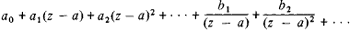# Laurent Series

(redirected from Laurent expansion theorem)

## Laurent series

[lȯ′ränz ‚sir·ēz]
(mathematics)
McGraw-Hill Dictionary of Scientific & Technical Terms, 6E, Copyright © 2003 by The McGraw-Hill Companies, Inc.
The following article is from The Great Soviet Encyclopedia (1979). It might be outdated or ideologically biased.

## Laurent Series

a series of the form(*)

that is, a series of positive and negative powers of the difference z — a (where z, a, and the coefficients of the series are complex numbers). Here, the nonnegative powers form an ordinary power series that converges, in general, within a circle with center a and radius R ≤ ∞. The remaining terms form a series that converges, in general, outside a circle with the same center but with radius r ≥ 0. If r < R, then the series (*) converges in the annulus r < ǀz — a < R to an analytic function of the complex variable z.

Although series of the form (*) were already known to L. Euler (1748), they were named after P. Laurent, who in 1843 proved that every function of a complex variable, single-valued and analytic in the annulus r < |z — | < R, can be expanded in this annulus in this type of series (Laurent theorem). The theorem, however, was obtained somewhat earlier by K. Weierstrass in a work that remained unpublished until 1894.